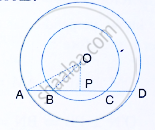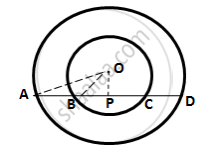Share

# In the Following Figure, Ad is a Straight Line. Op ⊥ Ad and O is the Centre of Both the Circles. If Oa = 34 Cm. Ob = 20 Cm and Op = 16cm; Find the Length of Ab. - Mathematics

Course

#### Question

In the following figure, AD is a straight line. OP ⊥ AD and O is the centre of both the circles. If OA = 34 cm. OB = 20 cm and OP = 16cm; find the length of AB.#### SolutionFor the inner circle, BC is a chord and OP⊥ BC.
We know that the perpendicular to a chord, from the centre of a circle, bisects the chord.

∴ BP = PC
By Pythagoras theorem,
OA2 = OP2 = BP2
⇒ BP2 = (20)2 - (16)2 =144
∴ BP= 12cm

We know that the perpendicular to a chord, from the centre of a circle, bisects the chord.
∴ AP = PD
By Pythagoras Theorem,
OA2 = OP2 + AP2
⇒   AP2 = (34)2 − (16)2 = 900
⇒    AP = 30 cm
AB = AP − BP = 30 − 12 = 18 cm

Is there an error in this question or solution?